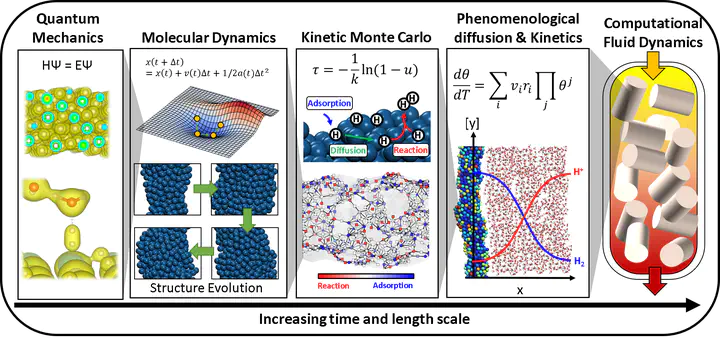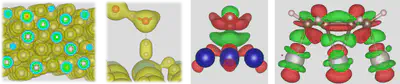# MultiScale Simulation StrategyThe time and length scale at which chemical phenomena occur can be categorized into several types. At the smallest scale that catalysis scientists consider, electrons rapidly equilibrate their orbital given the position of their nucleus which occurs at a time less than a picosecond. These atoms can vibrate around their bond length and molecules move around the space which constitutes thermodynamic properties that we calculate using statistical thermodynamics. These vibrating, translating, and rotating molecules can react together to form new molecules occurring at a time scale of about a picosecond. This set of reactions then changes the local concentration within, for example, a reactor at a time scale of a second. At a larger scale, the concentration gradient within a reactor needs to be accounted for via convection and diffusion. To account for these various phenomena that obey different physical laws, we need to use several tools in order to simulate the observable properties such as conversion, selectivity, etc.

## Quantum mechanics simulationQuantum mechanical simulation aims to solve the Shrodinger equation: HΨ = EΨElectrons and nuclei interact with each other under electrostatic interactions. Solving the Shrodinger equation can tell us the location and probability of electrons existing. By solving the Shrodinger equation, we can calculate the energy of a system. The properties of materials such as light adsorption and catalysis are determined by electronic interaction between atoms. Recent advancement in density functional theory (DFT) has enabled the solving Shrodinger equation with good accuracy. While the light adsorption properties and stability of the materials can be calculated with good accuracy, DFT cannot directly calculate the observable properties such as conversion, selectivity, etc. Thus, we need to implement additional tools to obtain some of the properties that scientists are interested in understanding.

## Molecular dynamics

At a larger scale, atoms in a molecule vibrate around their equilibrium position. The molecules rotate and translate freely in the case of gas and liquid states. DFT is usually used as a tool to calculate the ground state of the system. For example, water molecules form hexagonal layers when it becomes ice. However, as the temperature rises, the ice melts and the water structure becomes irregular. For these high entropy states, which is a system with a very large number of structural configurations with similar energy, calculating the ground state structure is not sufficient, but the motions of atoms need to be explicitly accounted for to understand the emergent properties of the high entropy system. The molecular dynamics simulates and tracks the individual motions of atoms at each time state, thus we can understand the emergent properties. See these great simulation videos done by scientists (video1, video2) for examples of water molecular dynamics simulation.

## Kinetic Monte Carlo and phenomenological kinetics

The kinetic Monte Carlo simulation of jagged Pt nanowire from Gu et al. JACS 2021
The vibration of atoms and rotation and translation of molecules occur rapidly and can be assessed via statistical thermodynamics to calculate the observable properties such as Gibbs free energy. The reactions, where molecules transform to a different chemical structure, occur much more slowly. In order to simulate reactions, the reaction barriers are assessed using quantum mechanics simulation and converted to reaction rate, typically using the transition state theory. The reaction subsequently changes the concentration of species in the system until the equilibrium is reached, the time scale of which can be about a micro second to hundreds of seconds. Kinetic Monte Carlo simulates individual elementary reactions whereas phenomenological kinetic models simulate the reaction of a species as a bulk. With Kinetic Monte Carlo, we can observe the change of individual molecules, thus we can observe the spatial interactions of molecules of a system. The phenomenological kinetic simulation sees a species as a whole, assuming that they are well-dispersed in the system and that the total reaction rate for each species is simulated. It is difficult to obtain an atomistic insight with phenomenological kinetics, but the simulations are often significantly faster, allowing us to understand a longer time scale. With kinetic simulation, we can connect the information obtained from quantum mechanics to the observable such as conversion and selectivity, from which we can obtain mechanistic insights and design principles.

## Computational fluid dynamics

The placement of the catalyst pallets in the reactor, the fluid characteristics, and the mixing affect the concentration gradient that exists in the reactor. While all catalyst pallets follow the kinetics based on their quantum mechanical characteristics, statistical thermodynamics, and kinetics, the gradients created by the fluid characteristics in the reactor create the concentration gradients, which change not just based on the kinetics, but also based on the diffusion and convection. Thus, to understand the final products of a reactor system that you would observe in the chemical plant, the fluid dynamics are simulated. See this video created by CPFD software.